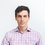# Expected variance in sample statistics

To start, let's calculate the mean of the binomial distribution, We expect of course, to find $$\langle \hat{A} \rangle = Np_A$$. According to our formula,

\begin{aligned} \langle \hat{A} \rangle &= f'(1) \\ &= \frac{\partial}{\partial z} \left(p_Az+p_B\right)^N\bigg|_{z=1} \\ &= N p_A \left(p_A+p_B\right)^{N-1} \\ &= N p_A \end{aligned}

And, so, the sample mean, $\langle\hat{A}\rangle$ is given by $Np_A$. So far, so good.

Now let's find the sample variance. For this, we'll need the additional piece $f''(1)$:

\begin{aligned} f''(z) &= \frac{\partial^2}{\partial z^2} \left(p_Az+p_B\right)^N \\ &= Np_A\frac{\partial}{\partial z} \left(p_Az+p_B\right)^{N-1} \\ &= N(N-1)p_A^2 \left(p_Az+p_B\right)^{N-2} \\ f''(1) &= N(N-1)p_A^2 \left(p_Az+p_B\right)^{N-2}\bigg|_{z=1} \\ &= N(N-1)p_A^2 \end{aligned}

With $f''(1)$ in hand, we find the sample variance

\begin{aligned} \sigma^2(\hat{A}) &= N(N-1)p_A^2 + Np_A - N^2p_A^2 \\ &= N^2p_A^2 - Np_A^2 + Np_A - N^2p_A^2 \\ &= Np_A(1-p_A) \end{aligned}

So that the sample standard deviation is $\sigma(\hat{A}) = \sqrt{Np_A(1-p_A)}$.

Recall that our purpose in doing all this was to calculate the uncertainty in our estimation of the true frequency. We can illuminate our results by writing them in terms of the sample frequency.

$\hat{p_A} = \hat{A}/N$, so, $\langle\hat{p_A}\rangle=\hat{A}/N=p_A$, and we expect on average for the sample frequency to equal the true frequency. In other words, if we take infinitely many subsets of the population and average all our sample frequencies, we will find the true frequency.

We can re-write the sample standard deviation $\sigma(\hat{A}) = \sqrt{\langle \hat{A}^2 \rangle - \langle \hat{A} \rangle^2} = N\sqrt{\langle p_A^2\rangle - \langle p_A \rangle ^2} = N\sigma(\hat{p_A})$ so the spread in our estimate of the true frequency (and the spread used by polling companies) is given by $\displaystyle\sqrt{\frac{p_A(1-p_A)}{N}}$, which is plotted below for several value of $N$.The uncertainty has several interesting features.

As we increase our sample size, we have, initially, big gains in our absolute certainty. However, as $N$ continues to increase, we get less and less additional certainty per unit investment in sample size. I.e. we have diminishing returns. Our results suggest that above $N\approx 1000$, it doesn't make much sense to continue building the sample size because the error is already about $1\%$ for all possible values of $p$. To cut that number in half, we'd have to increase our sample size by a factor of four to 4000 respondents.

$p(1-p)$ has a maximum at $\frac12$, therefore populations with true frequencies close to 0.5 produce the highest uncertainty in estimations, and extreme results produce the least. Likewise, if we consider $N$ as a cost, it is more expensive to establish a given level of certainty when $p$ is close to $\frac12$ than when it is close to zero or one. Here, we see the so-called TANSTAAFL principle at work (There Ain't No Such Thing As A Free Lunch).

When $p$ is far away from a coin flip, there is information-a-plenty and it is inexpensive to mine (we can achieve low uncertainty with smaller $N$). Close to $\frac12$ however, there is hardly any information in the system and so, it is much more expensive to unearth any of it.

Things get a bit more involved when questions have more than two choices, and we could treat confidence intervals in more detail, but that is poll uncertainty in a nutshell.

Before we wrap up, let's take a quick look at applications of the ideas we've explored in the realm of statistical physics.Note by Josh Silverman
7 years, 3 months ago

This discussion board is a place to discuss our Daily Challenges and the math and science related to those challenges. Explanations are more than just a solution — they should explain the steps and thinking strategies that you used to obtain the solution. Comments should further the discussion of math and science.

When posting on Brilliant:

• Use the emojis to react to an explanation, whether you're congratulating a job well done , or just really confused .
• Ask specific questions about the challenge or the steps in somebody's explanation. Well-posed questions can add a lot to the discussion, but posting "I don't understand!" doesn't help anyone.
• Try to contribute something new to the discussion, whether it is an extension, generalization or other idea related to the challenge.

MarkdownAppears as
*italics* or _italics_ italics
**bold** or __bold__ bold
- bulleted- list
• bulleted
• list
1. numbered2. list
1. numbered
2. list
Note: you must add a full line of space before and after lists for them to show up correctly
paragraph 1paragraph 2

paragraph 1

paragraph 2

[example link](https://brilliant.org)example link
> This is a quote
This is a quote
    # I indented these lines
# 4 spaces, and now they show
# up as a code block.

print "hello world"
# I indented these lines
# 4 spaces, and now they show
# up as a code block.

print "hello world"
MathAppears as
Remember to wrap math in $$ ... $$ or $ ... $ to ensure proper formatting.
2 \times 3 $2 \times 3$
2^{34} $2^{34}$
a_{i-1} $a_{i-1}$
\frac{2}{3} $\frac{2}{3}$
\sqrt{2} $\sqrt{2}$
\sum_{i=1}^3 $\sum_{i=1}^3$
\sin \theta $\sin \theta$
\boxed{123} $\boxed{123}$